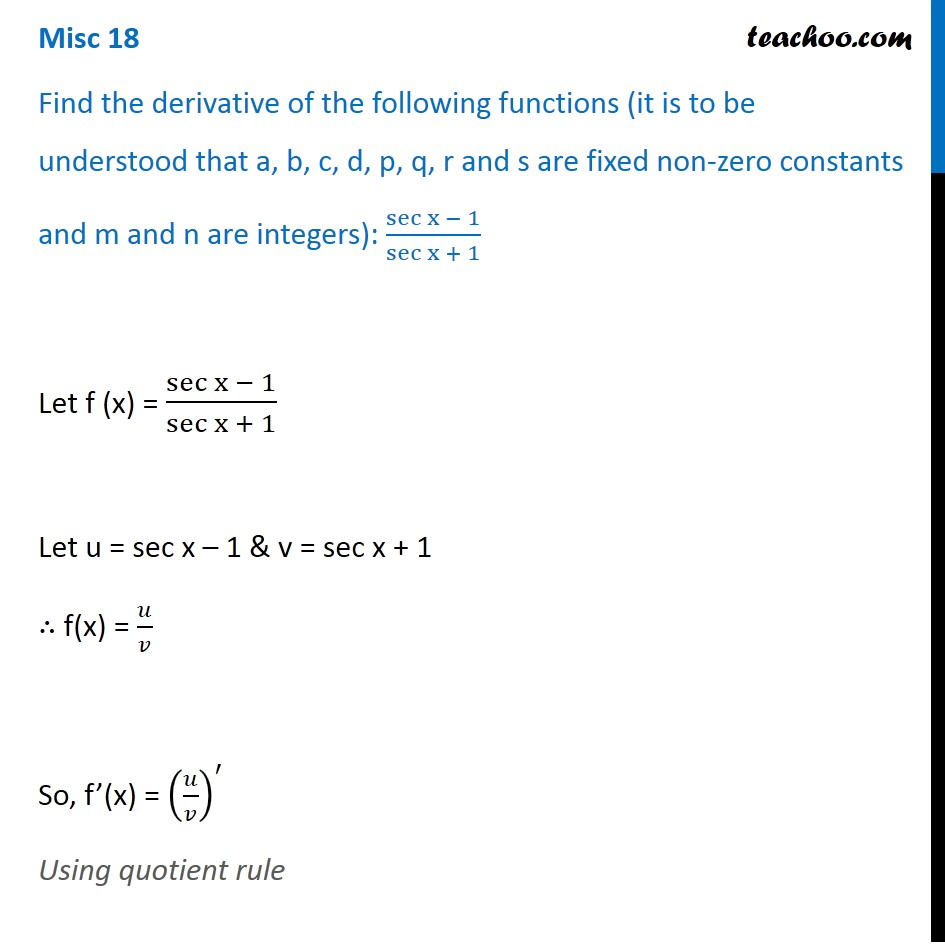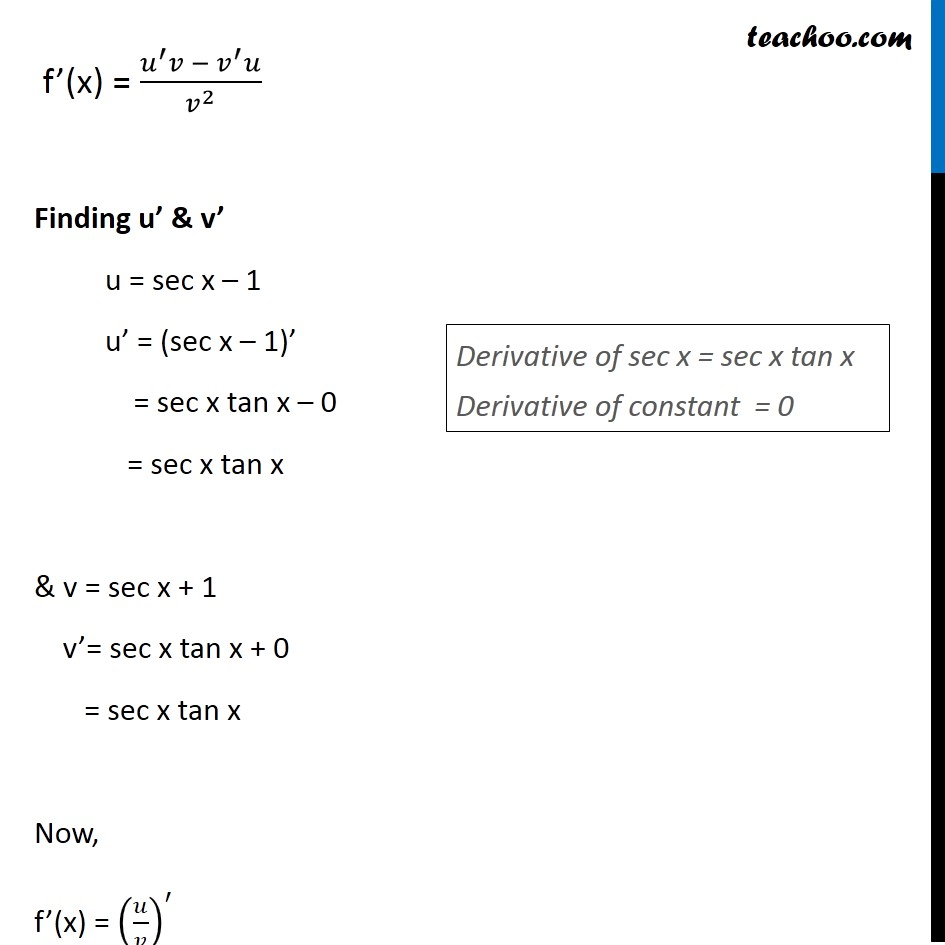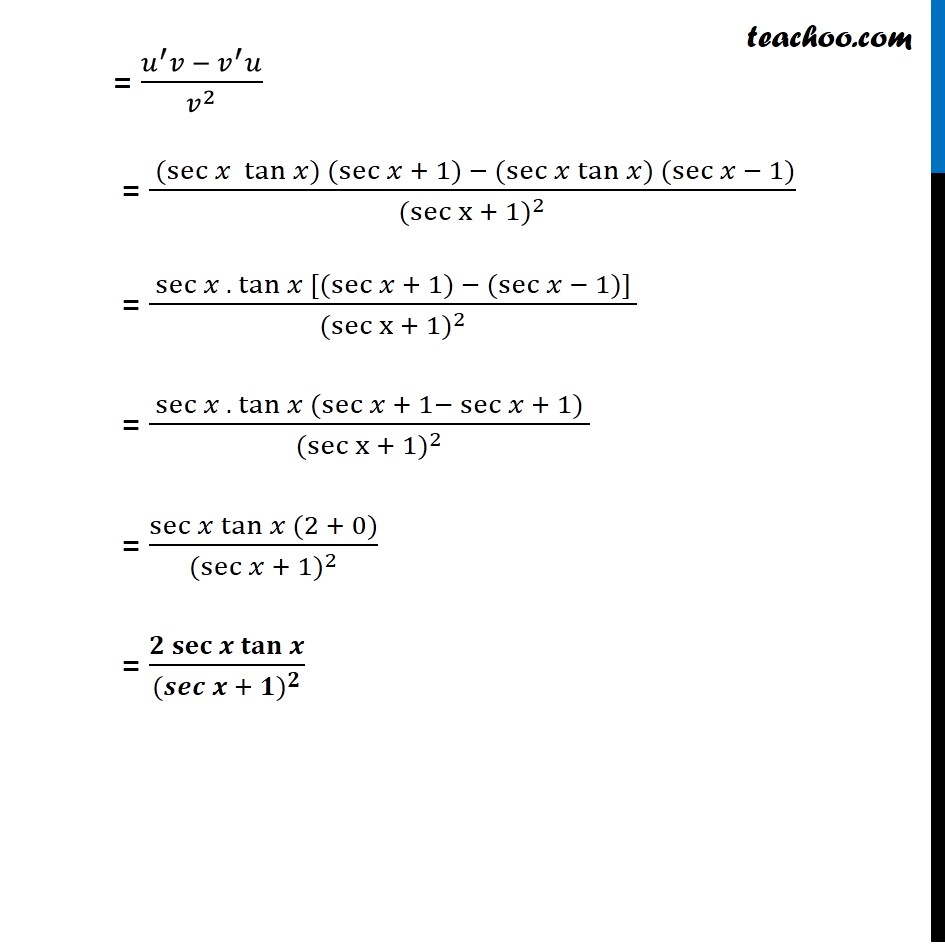Derivatives by formula - sin & cos

Chapter 12 Class 11 Limits and Derivatives
Concept wiseLearn in your speed, with individual attention - Teachoo Maths 1-on-1 Class

### Transcript

Misc 18 Find the derivative of the following functions (it is to be understood that a, b, c, d, p, q, r and s are fixed non-zero constants and m and n are integers): sec⁡〖x − 1〗/sec⁡〖x + 1〗 Let f (x) = sec⁡〖x − 1〗/sec⁡〖x + 1〗 Let u = sec x – 1 & v = sec x + 1 ∴ f(x) = 𝑢/𝑣 So, f’(x) = (𝑢/𝑣)^′ Using quotient rule f’(x) = (𝑢^′ 𝑣 −〖 𝑣〗^′ 𝑢)/𝑣^2 Finding u’ & v’ u = sec x – 1 u’ = (sec x – 1)’ = sec x tan x – 0 = sec x tan x & v = sec x + 1 v’= sec x tan x + 0 = sec x tan x Now, f’(x) = (𝑢/𝑣)^′ Derivative of sec x = sec x tan x Derivative of constant = 0 = (𝑢^′ 𝑣 −〖 𝑣〗^′ 𝑢)/𝑣^2 = ( (sec⁡〖𝑥 tan⁡〖𝑥)〗 (sec⁡〖𝑥 + 1) − (sec⁡〖𝑥 tan⁡〖𝑥)〗 (sec⁡〖𝑥 − 1)〗 〗 〗 〗)/〖(sec⁡〖x + 1〗)〗^2 = ( sec⁡〖𝑥 . tan⁡𝑥 [(sec⁡〖𝑥 + 1) − (sec⁡〖𝑥 − 1)] 〗 〗 〗)/〖(sec⁡〖x + 1〗)〗^2 = ( sec⁡〖𝑥 . tan⁡𝑥 (sec⁡〖𝑥 + 1−〖 sec〗⁡〖𝑥 + 1〗) 〗 〗)/〖(sec⁡〖x + 1〗)〗^2 = sec⁡〖𝑥 tan⁡〖𝑥 (2 + 0)〗 〗/〖(sec⁡〖𝑥 + 1〗)〗^2 = (𝟐 𝐬𝐞𝐜⁡〖𝒙 𝐭𝐚𝐧⁡𝒙 〗)/〖(𝒔𝒆𝒄⁡〖𝒙 + 𝟏〗)〗^𝟐